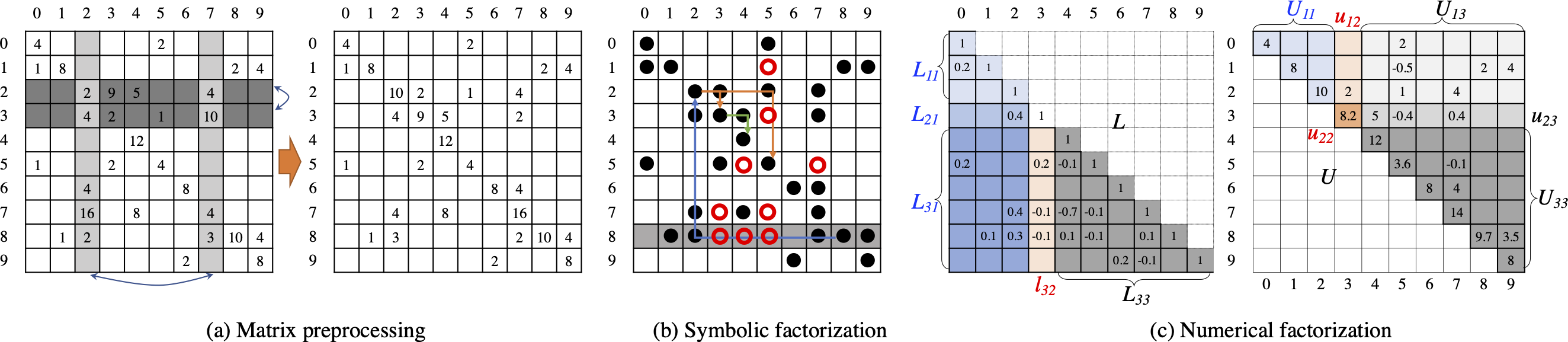## Scaling LU Factorization beyond 1,000 GPUsProject Description: Many scientific and engineering problems require solving large-scale linear systems, Ax = b. Solving this problem with direct methods often involves LU factorization, that is, decomposing the original matrix A into lower and upper triangular matrices L and U, respectively, where A = LU. Since LU decomposition of a sparse matrix typically introduces more nonzeros, also referred to as fill-in, than the original matrix A, symbolic factorization is designed to compute the structure of these nonzeros for both L and U. This information is subsequently used to predict the essential memory space for the subsequent numerical factorization. This project aims to develop a scalable symbolic factorization algorithm on GPUs which are absent in current practice.

• Personnel:
• Hang Liu (PI)
• Xiaoye S. Li (Collaborator)
• Anil Gaihre (PhD student)
• Luca Pasquariello (Master student)
• Zehui Xie (Master student)
• Publications:
• Acknowledgement: